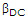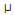# Electronics - Bipolar Junction Transistors (BJT) - Discussion

### Discussion :: Bipolar Junction Transistors (BJT) - General Questions (Q.No.3)

3.

A transistor has aof 250 and a base current, IB, of 20A. The collector current, IC, equals:

 [A]. 500A [B]. 5 mA [C]. 50 mA [D]. 5 A

Answer: Option B

Explanation:

No answer description available for this question.

 Koudinya said: (Oct 19, 2010) Ic = Beta x Ib

 Laxman said: (Dec 3, 2010) Ic=Beta*Ib Ic=250*20/1000000 =5000/1000000 Ic=5mA

 Rajnish Tiwari said: (Dec 11, 2010) Beta=Ic/Ib Ic=Beta*Ib Ic=250*20/1000000 Ic=5mA

 Arghya Das said: (Jan 18, 2011) Beta is 250,Ib=20 collector current Ic=(Ib*beta)/10^6 Ic=5000/10^6 =5mA

 Sashibhusan Panda said: (Sep 18, 2011) For dc operation of bjd Ic=Ie+(beta)Ib But for Ib=20ua current value, Ie negligible so considered as zero. => Ic=250*20uA=5mA. (ans)

 Micheal said: (Oct 7, 2011) Bdc is 250 and current base Ib=20 so they formula say that collector current(Ic)= Ib*Bdc then IC= 20*250 =5000 sol: 5mA

 Jyothi said: (Nov 10, 2011) Ic=BIb =250*20*10^-6 5 mA

 Shreshthagupta said: (Feb 27, 2012) Ic=Beta*Ib =250*20*10^-6 =5*10^-6 =5mA

 Bala.J said: (Apr 20, 2012) THE FORMULA USED TO FIND OUT THE IC IS Ic = Ib*GAIN = (20*10^-6)*(250) =5*10^3* 10^-6 = 5mA

 Kalpana said: (Sep 29, 2012) In common emiter configuration, amplification factor is given by BETE=Ic/Ib. Ic=BETA*Ib =250*(20*10^-6) =5000*10^-6 =5mA

 Sid said: (Jan 1, 2013) From formula, Ic(collector current) = Beta*Ib(base current). =250*20*10^-6. =5*10^-3. =5mA.

 Tejaswini said: (Aug 4, 2013) Ic = BIb. Ic = 250*20*10^-6. Ic = 5mA.

 Dieudonne Kubwimana said: (Apr 18, 2017) Given datas:bette = 250,current base = 20micro ampere. Then IC = bette*current base. IC = 250 * 20 = 5000micro ampere. Convetion of 5000MA into mA will be 5mA(Ans).

 Max Malick Electronic said: (Feb 7, 2018) β = Ic/Ib. Ic=betta * Ib. Ic = 250 * 20micro Ampere. Ic = 5mA.

 Moin Ansari said: (Aug 25, 2018) As we know that the amplification factor in ce mode β=Ic/Ib. and Ib is in micro range so 20*1000000, 250*20/1000000=5ma answer.

 Deepen said: (Nov 12, 2018) You are right, Agree @Kalpana.

#### Post your comments here:

Name *:

Email   : (optional)

» Your comments will be displayed only after manual approval.

#### Current Affairs 2021

Interview Questions and Answers